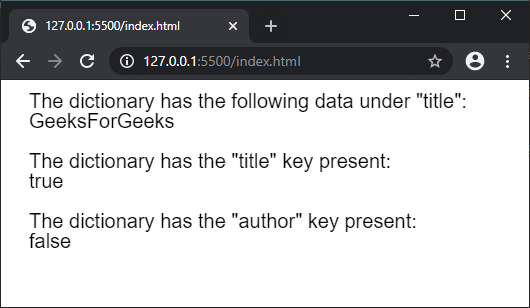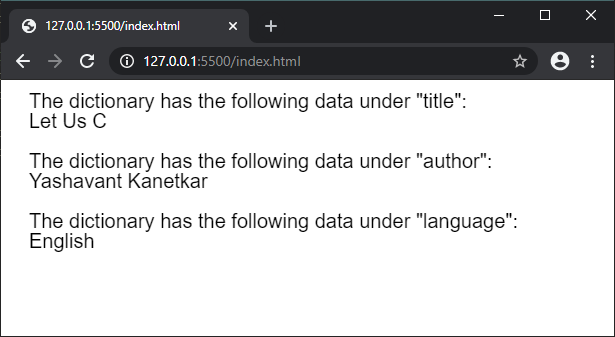# p5.js | createStringDict() Function

• Last Updated : 20 Aug, 2021

The createStringDict() function is used to create a p5.StringDict instance with the given data. The data can be individually passed as a key-value pair or given as a collection of values using an object.

Syntax:

`createStringDict(key, value)`

or

`createStringDict(object)`

Parameters:

• key: It specifies the string that is used as the key in the dictionary.
• value: It specifies the string that is used as the value in the dictionary.
• object: It specifies the object that is used as the dictionary.

Return Value: It returns a p5.StringDict object with the given data.
The program below illustrate the createStringDict() function in p5.js:
Example 1:

## javascript

 `function` `setup() {``  ``createCanvas(500, 200);``  ``textSize(20);` `  ``// Creating a string dictionary``  ``// with the given key and value pair``  ``let mydict = createStringDict(``"title"``, ``"GeeksForGeeks"``);` `  ``// Accessing the data using the data property``  ``text(``'The dictionary has the following data under "title":'``, 20, 20);``  ``text(mydict.data.title, 20, 40);` `  ``// Checking if a key exists in the dictionary``  ``text(``'The dictionary has the "title" key present:'``, 20, 80);``  ``text(mydict.hasKey(``"title"``), 20, 100);` `  ``text(``'The dictionary has the "author" key present:'``, 20, 140);``  ``text(mydict.hasKey(``"author"``), 20, 160);``}`

Output:Example 2:

## javascript

 `function` `setup() {``  ``createCanvas(600, 200);``  ``textSize(20);` `  ``let obj = {``    ``book: ``"Let Us C"``,``    ``author: ``"Yashavant Kanetkar"``,``    ``language: ``"English"``  ``}` `  ``// Creating a string dictionary``  ``// with the above object``  ``let mydict = createStringDict(obj);` `  ``// Accessing the data using the data property``  ``text(``'The dictionary has the following data under "title":'``, 20, 20);``  ``text(mydict.data.book, 20, 40);``  ` `  ``text(``'The dictionary has the following data under "author":'``, 20, 80);``  ``text(mydict.data.author, 20, 100);``  ` `  ``text(``'The dictionary has the following data under "language":'``, 20, 140);``  ``text(mydict.data.language, 20, 160);``}`

Output:Online editor: https://editor.p5js.org/
Environment Setup: https://www.geeksforgeeks.org/p5-js-soundfile-object-installation-and-methods/
Reference: https://p5js.org/reference/#/p5/createStringDict

My Personal Notes arrow_drop_up